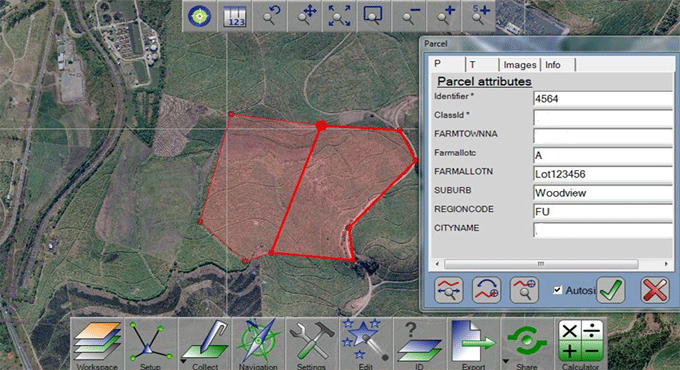# How to measure the land area for irregular & large land tractsVarious types of surveying methods are used for conducting survey of irregular and large land tracts. Some methods are easily understandable and based on elementary geometry, others are complicated and may contain GPS and satellite imagery.

In this article, useful information is given on various options and technologies accessible to the surveyor.

The calculation should be done for Land for different purposes like prior to purchase, while performing stock taking, erecting a boundary wall, controversy with a neighbor over demarcation, etc. Making calculation for regular land area is simple since they contain mostly rectangle or other geometrical shapes. Recalling geometry studied in High School we can do the measurements and arrive at the results. But, the process becomes difficult for measurement, if the area of land is extremely irregularly shaped or large tracts of land are purchased like a farm measuring miles across.

In this article, you will be familiar with some self-reliant methods of calculating your land and some high technology solutions.

Given below, some useful methods for measuring the land with simple geometry, maps and planimeter, GPS, Aerial photography, Satellite imagery, Google earth, maps and graphs, measuring wheels.

Geometric Method: Under this method the odd shaped plot is surveyed and plotted over graph paper. The area of the plot is then subdivided into geometric figures and the areas of these figures are measured with formulae.

Initially, the greater size geomatric figure that can be perfectly drawn in the map is created and after that smaller shapes are produced unless the complete space is captured more or less.

Once the computation for areas of these figures is done, then these are added to determine the total area.

As for instance:
Area of Rectangle: Length x Breadth
Area of Square: Length x Length
Area of Triangle: 0.5 x Base x Height
Area of Trapezium: 0.5 x (Length 1 + Length 2) x Height
The above formulas are used to find out the exact area.

Planimeter on Map: Planimeter is a small gadget that is utilized for working out the areas of a graphically represented planar region. The planimeter can calculate all regular and irregular shapes. It is required to pivot it at any point and then run the end pin all over the perimeter of the area to be calculated. It contains a compliant linkage that facilitates it to move devoid of any effort in all directions.